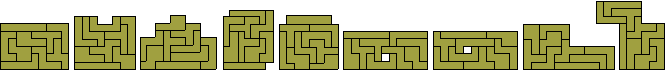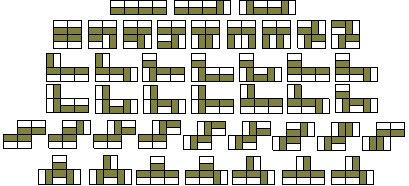An n-are is a shape formed from an n-omino by the removal of half of each square in such a way that at least half of the original join between squares is retained.The 9 triares formed from the two trominoes

Below are six constructions made with this set of pieces. The only rectangle possible is the 6x9 shown. There are a number of solutions to a 5x11 rectangle with a single hole. This hole cannot be at the centre of the rectangle because of colouring considerations. The constructions at the right are triplications of two of the pieces. It is likely that the other triplications are not possible.The 42 tetraresThe 42 tetrares cover a total area of 84 full squares and could therefore cover rectangles 2x42, 3x28, 3½x24, 4x21, 6x14, 7x12, 8x10½. The 2x42 is not possible as can be seen from the fact that at least one of the pieces (below) must divide the rectangle into parts whose areas are not multiples of two unit squares.The diagram below shows two 3x14 rectangles and two 4x10½ rectangles which can combine to form the 3x28, 4x21, 6x14, and 8x10½ rectangles.There is also a number of other constructions which could be possible with the set such as the two squares below.The tetrares are very difficult to work with and even using only 36 pieces to form a sextuplication of one of the pieces is no easy task. One example of this is shown below.Pentares

There are 180 pentares including two pieces with holes. My thanks to Brendan Owen for checking the number of pentares and for providing a diagram of the hexares and the following table
 n Number of n-ares Number of one-sided n-ares 1 1 1 2 3 4 3 9 16 4 42 77 5 180 351 6 889 1747 7 4392 8745 8 224332 44724

One-sided polyares

If we consider mirror images as distinct there are 16 triares with a total area of 96 units. With this set 4x24, 6x16 and 8x12 rectangles are all possible as shown below where there are two 4x12 rectangles made and two 6x8 rectangles from the set. Also included are two constructions based on a 10x10 square.The two 4x12 rectangles also provide a solution to the problem of quaruplication for two of the pieces. All quadruplications are possible. The remainder are shown below.There are 77 one-sided tetrares which could form the figure below.